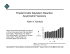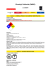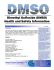# Molar refractivity and interactions in solutions IL Molar refractivity of

## Transcription

Molar refractivity and interactions in solutions IL Molar refractivity of
```Molar refractivity and interactions in solutions
IL Molar refractivity of tetraalkylammonium salts in aqueous
and dimethyl sulfoxide solutions
P. PACÁK
Institute of Inorganic Chemistry, Czechoslovak Academy of Sciences,
CS-160 00 Prague
The density and refractive index were determined in aqueous and dimethyl sulfoxide solutions of some tetraalkylammonium chlorides, bromides, iodides, and nitrates. The molar refractivities of the individual ions
were estimated and the polarization effect of the anions on the solvent is
discussed.
Определены величины плотности и показателя преломления рас­
творов хлоридов, бромидов, иодидов и нитратов нескольких тетраалкиламмониевых катионов в воде и диметилсульфоксиде. Определены
значения молярных показателей преломления отдельных ионов и оха­
рактеризован поляризационный эффект анионов на растворитель.
The molar refractivity reflects the changes in the properties of the ions due
to polarization or deformation of their electron shells under the influence of the
electric field of neighbouring ions. The refractivity values of individual ions in
the free gaseous state [R]g calculated by Pauling  and Fajans  serve as a
measure of their respective deformabilities.
In the previous paper , the molar refractivities of some salts were studied
in aqueous and dimethyl sulfoxide solutions and individual ionic refractivities
at infinite dilution were estimated. Ions exert no forces upon one another in
infinitely dilute solutions and the change in the ionic refractivity accompanying
transition from the gaseous state to infinite dilution is a measure of ion—solvent
interactions. It was found that chloride and nitrate anions did not influence
water molecules  whereas both the anions exert a polarization effect on
dimethyl sulfoxide, which caused a decrease in the solvent refractivity .
The refractivity of aqueous and dimethyl sulfoxide solutions of tetraalkylam­
monium salts was studied in this contribution in order to confirm the polariza­
tion effect of the anions on the solvent. It is believed that the large cations do
not influence the solvent molecules, so the refractivity change in the studied salts
can be ascribed to the effect of the anion alone.
Chem. Papers 43 (4) 501—505 (1989)
501
P. PACÁK
Experimental
Tetraalkylammonium chlorides, bromides, and iodides (anal, grade, Merck) were
used as received. The purity of the salts was controlled by Volhard titration for anion
content and it was always more than 99 %. Dimethyl sulfoxide — DMSO (anal, grade,
Merck) containing less than 0.03 % water was used without further treatment.
Tetramethyl- and tetraethylammonium nitrates were prepared from a 10 % solution
of the respective hydroxides by neutralization with HN03 at 10 °C. Water was then
removed at 60 °C using a vacuum rotatory evaporator. A concentrated solution of the
nitrate salt crystallized at 5 °C and, after filtration, was washed with acetone and dried
at 60 °C in a vacuum oven. The amount of nitrate anions in the sample was determined
spectrophotometrically  as 99.6%.
The determination of the density and the refractive index was described earlier . The
total error in the molar refractivity determination was estimated as ±0.05 cm3 mol-1 It
should be noted that the accuracy of the calculated salt molar refractivity decreases with
decreasing content of the salt in the solution. At x < 0.05, the error may be higher than
±0.1 cm3 mol-1 The composition of the solutions is expressed in terms of the mole
fraction of the salt x.
The measurements were carried out at 30 and 45 °C in DMSO and aqueous solutions,
respectively. As the dependence of the molar refractivity on the temperature is less than
3 x 10_4cm3mol_I K 1 , the two values measured at 30 and 45°C can be compared.
All the refractivity values are referred to a wavelength of 586.7 nm (He, D-line). The
numerical values of the density and the refractive index are available from the author
upon request.
Results and discussion
The molar refractivity of the solution [R]soln (cm3 mol"1) was calculated using
the Lorenz—Lorentz equation
L^Jsoin
=
"~;2
7 * Kn
n + 2
where n is the refractive index and Vm is the molar volume of the solution. The
molar refractivities of the salt [Rx] were calculated for various amounts of salt
by the method described in the previous paper  using the refractivity values
of the pure solvents from Ref. . The dependence of [Rx] on the mole fraction
of salt is depicted in Fig. 1 and the refractivity values at infinite dilution [R°]
were estimated by extrapolation to the value of x = 0. The values of [R°] are
listed in Table 1 both for aqueous and DMSO solutions. The concentration
dependence of the molar refractivity was measured up to saturated solutions of
the salts. The empty spaces in Table 1 indicate that the salt was less soluble in
a given solvent than would correspond to x = 0.025.
502
Chem. Papers 43 (4) 501—505 (1989)
MOLAR REFRACTIVITY II
Fig. 1. The molar refractivity of
the salt [Rx] in dependence on
the mole fraction of the salt.
a) <g> (C 2 H 5 ) 4 NBr—DMSO;
x (C 2 H 5 ) 4 NBr—H 2 0;
Л (C 2 H 5 ) 4 NN0 3 —H 2 0;
D(C 2 H 5 ) 4 NN0 3 —DMSO;
О (C 2 H 5 ) 4 NC1—H 2 0;
• (C2H5)4NC1—DMSO.
b) • (CH 3 ) 4 NN0 3 —H 2 0;
О (C 4 H 9 ) 4 NI—DMSO;
[*x3
W
& Ц)"
H-
cm mol
51
49
33
" • • v^«
98
97
zx
31
96
30 0.1
0.2
Table 1
Refractivities of salts in infinitely dilute aqueous [Ä°]H20 and DMSO [Ä°]DMSO solutions (cm3 mol - ')
Salt
[R°lIH20
(CH3)4NC1
(CH 3 ) 4 NN0 3
(C2H5)4NC1
(C2H5)4NBr
(C2H5)4NN03
(C4H9)4NI
31.1
32.9
48.26
52.2
50.36
[R°)D
47.9
52.1
49.78
96.83
The refractivity values of the chloride and nitrate anions in infinitely dilute
aqueous and DMSO solutions [3,4] are listed in Table 2. Using these values, the
refractivity of the cations was evaluated assuming the additivity of the ionic
refractions in infinitely dilute solutions (Table 3).
Chem. Papers 43 (4) 501—505 (1989)
503
P. PACÁK
Table 2
Refractivities of anions in infinitely dilute solutions in H 2 0 and DM SO (taken from Ref. [3, 4])
Anion
[R°
m9.06
H 20
c\-
8.61
12.67°
19.22
10.38
12.67
19.22
11.02
I"
N0 3 a) The result of this work.
Table 3
Refractivities of tetraalkylammonium cations in infinitely dilute solutions in H 2 0 and DM SO
calculated from the equation [/?° J = [Ä°iJ — [Amnion]- (The refractivity values of anions are given in
Table 2.)
[Ä°]H20/(cm3 mol "')
Cation
+
(CH3)4N
(C2H5)4N+
(C4H9)4N+
[^loMso/ícm^ol- 1 )
СГ
Br"
N0 3 -
c\-
Вг"
N0 3 -
22.04
39.2
39.5
21.9
39.34
39.3
39.4
39.4
I"
77.6
The molar refractivity reflects the dispersivity of the valence electrons which
depends on the mass, charge, and polarizability of the molecule. As an increas­
ing number of electrons take part in the bonding the molecule becomes more
readily deformable. Thus, we can expect the refractivity values of the tetraalkyl­
ammonium cations to be higher than those of the simple univalent cations .
2
3
carbon atoms
504
Fig. 2. The molar refractivity of the te­
traalkylammonium cations in infinitely di­
lute solution [Ä° J in dependence on the
number of С atoms in the compound.
Chem. Papers 43 (4) 501—505 (1989)
MOLAR REFRACTIVITY. II
The refractivities of tetraalkylammonium bromides in infinitely dilute solu­
tions are almost equal both in aqueous and DMSO solutions whereas the
refractivity values differ for chloride and nitrate salts (Table 1). None of the
studied anions influences the water molecule  but chloride and nitrate anions
exert a polarizing effect on DMSO , which causes a decrease in the salt
refractivity. It follows that the bromide anion does not influence the DMSO
molecule and it is more than probable that the larger iodide anion will also have
no effect on DMSO, as was assumed in the previous paper .
The calculated refractivity values of the cations (Table 3) were found to be
mutually consistent in both solvents within the measuring error. This confirms
the refractivity values of chloride and nitrate anions in infinitely dilute aqueous
and DMSO solutions estimated earlier .
The dependence of the refractivity of the cations on the number of С atoms
is depicted in Fig. 2. The molar refractivity shows constant differences for equal
increments of a — C H 2 group, similarly as in homologous series of aliphatic
alcohols, acids, and esters .
References
1.
2.
3.
4.
5.
6.
7.
Pauling, L., Proc. R. Soc. London, A 114, 181 (1927).
Fajans, K. and Joos, G., Z. Phys. 23, 1 (1924).
Pacák, P., Chem. Papers 43, 489 (1989).
Fajans, K. and Luhdemann, R., Z. Phys. Chem. 29, 150 (1935) and references cited therein.
Malá, J., Novák, J., and Sláma, L, Chem. Listy 75, 655 (1981).
Pacák, P., J. Solution Chem. 16, 71 (1987).
Partington, J. R., Advanced Treatise on Physical Chemistry, Vol. 4, p. 43. Longmans, London,
1960.
Translated by P. Pacák
Chem. Papers 43 (4) 501—505 (1989)
505
```

### Molar refractivity and interactions in solutions I. Molar refractivity ofwhere a is the refractivity of the pure solvent taken from the work of Pacák . The refractivities of the solution were recalculated for selected concentrations using the regression constants and...

### Inversion for atmosphere duct parameters using real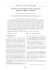### Efforts in Assimilation of Satellite data at NCMRWF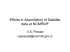### DMSO or DIMETHYL SULFOXIDE b y: Walter Last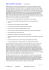### DuraSIL-SL - Best Materials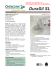### american museum novitates - AMNH Library Digital Repository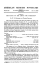### A Quick Fix for Pseudo-Class III Correction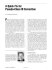### Quiz 5 Key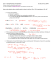### Multipod product flyer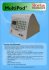### Friedel-Crafts Alkylation Reaction Asymmetric